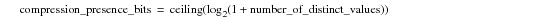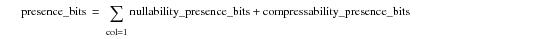# 15.00 - Presence Bits - Teradata Database

prodname
vrm_release
15.00
category
User Guide
featnum
B035-1094-015K

## Presence Bits

Presence bits indicate the status of each column with respect to its nullability, multi-value compressibility, and autocompressibility. Compression presence bits are added to the row header of each row to specify how multi-value compression is used for that row.

Each row has at least one octet of presence bits and can have more, depending on the degree of the table and the cumulative number of values compressed. The difference between bytes and octets is conceptual. The 8 bits of a byte define an atomic unit, while each individual bit of an octet is an atomic flag in a bit array. For the purpose of storage capacity analysis, both are treated as bytes. All rows for a given table have the same presence bits defined because nullability and multi-value compressibility are table attributes.

The first bit of the first presence bits octet is always set to 1, so the first octet defines the nullability and multi-value compressibility for no more than seven columns. When necessary, additional presence bit octets are added to the row header. Although only 7 of the bits in the first octet are available for use as presence bits, all 8 bits of successive octets are available. Eight bits are used because 255 compressed values plus null require 28, or 256 bit combinations, to be represented.

For a column‑partitioned table or join index there is one set of presence bits for each column partition value in the container. If a presence bit is 1, a value is present. If a presence bit is 0, no value is present.

A compression‑enabled column for a table has a maximum of 256 presence bits set, depending on how many values are compressed using multi-value compression.

The meaning of the number of presence bits set per column for compression of a single value is provided in the following table.

 This type of presence bit field… Has this many presence bits … For this type of column … Nullability 0 Non-nullable 1 Nullable Compressibility 0 Not compressed or compressed on nulls only. 1 Compressed on a value

For multi-value compression, the following equation calculates the number of compression presence bits required to define compression for a column in its table header.where:

 This term … Specifies … ceiling(x) a function that returns the smallest integer greater than or equal to x. This function is common in the mathematics library of most programming languages. See SQL Functions, Operators, Expressions, and Predicates for documentation of the Teradata SQL implementation of the ceiling function, CEIL. The ceiling function rounds the result of the expression up to the nearest integer, which is the exact number of presence bits required to account for both single‑valued and multi-value compression information for the given table. number_of_distinct_values the number of distinct values to be compressed for the column using multi-value compression.

The total number of presence bits for a given row is the sum of the nullability presence bits and the compressibility presence bits.The following table expresses the same information in a slightly different way.

 Compressible Nullable Bit Value Meaning Bit Value Meaning 0 The column is multi-value compressed. 0 The column is null. 1 A non-compressed column value is present. 1 The column is not null. An algorithmically compressed column adds an extra bit to indicate whether the column is algorithmically compressed or not, as follows: Bit Value Meaning 0 The column is not algorithmically compressed. 1 The column is algorithmically compressed. 1 to 8 bits for each multi-value compressible column (8 bits because 255 compressed values plus null require 28, or 256 bit combinations, to be represented). 1 bit for each nullable column.

The following table provides a comprehensive mapping of the presence bits and their various combinations for the multi-value compression case:

 WHEN the presence bits for a column have these values … THEN the column … AND … COMPRESS NULL no bit no bit is not compressible is not nullable. 0 no bit is compressed is not nullable. 1 no bit contains uncompressed column values is not nullable. no bit 0 is not compressible is null. no bit 1 is not compressible is not null. 0 0 is compressed is null. 1 1 is not compressed is not null. 1 0 is not compressed is null. 0 1 is not compressed. is null.

Mappings of COMPRESS bit values for multi-valued compression generalize from this specific case as illustrated by the following table.

 IF the presence bit is … THEN the data is … 1 not compressed. The corresponding compress bits are all 0. 0 compressed. If the corresponding compress bits are all 0, then the compress value is null. If the corresponding compress bits are not all 0, then the compress value is an index to the compress multi-value array in the table header.

The following table presents a set of examples that clarifies the correspondence between presence bits and data attribute specifications.

 FOR this column definition … The presence bits are … For these characters … `col_1 CHAR(1) NOT NULL` none A `col_1 CHAR(1) NOT NULL COMPRESS (‘A’)` 0 A 1 B `col_1 CHAR(1) COMPRESS` 0 null 1 A `col_1 CHAR(1) COMPRESS (‘A’)` 00 null 01 A 10 B 10 C `col_1 CHAR(1) COMPRESS (‘A’, ‘B’, ‘C’, ‘D’)` 0000 null 0001 A 0010 B 0011 C 0100 D 1000 E `col_1 CHAR(1) NOT NULL COMPRESS (‘A’, ‘B’, ‘C’, ‘D’)` 001 A 010 B 011 C 100 D 000 E